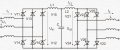# Stuck at finding out reactive power of a three phase DC power transmission system.

#### Anex

Joined Dec 4, 2017
7
So i've gotten so far as to calculate the rectifier delay angle α1 (36.79 deg) and the inverter delay angle α2(119.74 deg), with the givens being :
Phase voltage on the first side U1f(100kV), line voltage U1l(173kV) and second side U2f(127kV) and U2l(220kV), the frequency of both three phase systems f1 and f2 (f1=f2=50hz), the inductance on the first side of the three phase system Xa1(21 ohms) and Xa2(31 ohms) for second side, as well as the transferable voltage Ud(171kV) and current Id(800 A)
I'm supposed to calculate the reactive power and can't find the formula for it.
I'm thinking here that it might be something like this (i might be wrong though):
1. calculate active power Pa = 3*Ud*Id*cos(α1)
2. calculate full power S = 3*U1f*I1rms
3. calculate reactive power Q = $$f=(S^2-P^2)^\frac12$$
Or maybe im supposed to do an integral of U1f*cos(α) and I1rms from α to α+pi/3 to get the active power, honestly i'm a little bit stumped over here.
Picture of the DC transmission schematic (the inductor L1 and capacitor C in the middle can be ignored) :Any help would be appreciated!

Last edited: